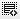Excel formulas0
Let me know the few more excel formulas?1

COUNT
Formula: =COUNT(A1:A10)

The count formula counts the number of cells in a range that have numbers in them.

LEN
Formula: =LEN(A1)

The LEN formula counts the number of characters in a cell. Be careful though! This includes spaces.

TRIM
Formula: =TRIM(A1)

Gets rid of any space in a cell, except for single spaces between words.

NOW Returns the system date and time

TODAY Returns the system date, without the time

SUMIF Calculates a sum from a group of values, but just of values that are included because a condition is met

COUNTIF Counts the number of cells in a range that match a criteria0

You can find more formulas infact all the formulas in excel itself.

From the menu bar select "FORMULAS" and then choose your formula which is categorised in various headings.

Click on thisicon to add code snippet.